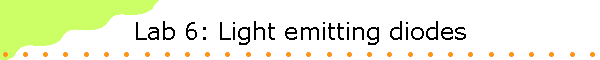In this laboratory you will measure the voltage across several visible light-emitting diodes (LED's) as a function of the current flowing through the diodes.  You will use your data to estimate the band gap of the semiconductor material the diode is made of and predict the wavelength of the emitted light.  You will check their predictions by measuring the wavelength of the peak in the diodes emission spectrum with a "Red Tide" spectrometer.Background information:

A light-emitting diode is a p-n junction rectifier.  When p- and n-type semiconductors are brought together to form a p-n junction, electrons with energies in the conduction band diffuse from the n-side to the p-side and holes with energies in the valence band diffuse from the p-side to the n-side.  Without an externally applied voltage, a diffusion potential VD is generated in the depletion layer between the n-type and the p-type material.  The diffusion potential prevents more electrons and holes from leaving the n- and p-regions, respectively, and entering the opposite regions.  When an external forward biased voltage V is applied, the potential  barrier is reduced.  When V ~ VD the height of the barrier is approximately zero and electrons can flow from the n-side to the p-side.  Recombination of electrons and holes now can continue and a current will flow across the junction from the p-type to the n-type material.  During the recombination energy is released.  It can be released in the form of  a photon with energy hf ~ Eg, where Eg is the  width of the bad gap of the semiconductor.

If we measure the minimum voltage Vmin required to cause photon emission, and we measure the wavelength of the emitted photons and use it to calculate the photon energy hf, we always find that eVmin < hf.  Some of the photon energy is supplied by thermal energy.  In order to predict the wavelength of the photons emitted by a LED, we must take into account the distribution of charge carriers in the semiconductor material.  The peak emission is expected at a photon energy somewhat higher than the Eg.

The anatomy of a common LED is shown below.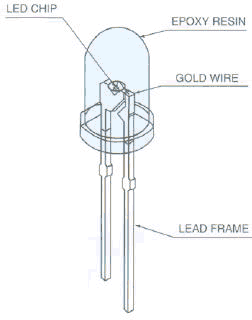The circuit symbol is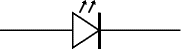Forward bias requires that the voltage at the anode (a) of the LED  is positive with respect to the voltage at the cathode (k).  The cathode has the shorter lead.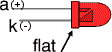Caution:  Never connect an LED directly to a battery or power supply!
It will be destroyed almost instantly because too much current will pass through and burn it out.  LEDs must have a resistor in series to limit the current to a safe value.  A 1 kΩ resistor is suitable for most LEDs if the supply voltage is 12V or less.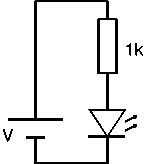The color of the plastic case around a LED chip does not always indicate the color of the emitted light.

Equipment needed

• PASCO PI-9877 DC Power Supply
• 1 kΩ resistor
• 2 digital multi-meters (DMMs) and test leads with small alligator clips
• Several LEDs
• Ocean Optics Red Tide Spectrometer

The table below lists the physical properties of typical LEDs.

Physical Properties of the LEDs
 Red Diode Yellow Diode Green Diode Blue Diode Wavelength (nm) 665±15 590±15 560±15 460±15 Color Red Yellow Green Blue Composition GaAs.6P.4: N GaAs.15P.85: N GaP:N InGaN

Procedure: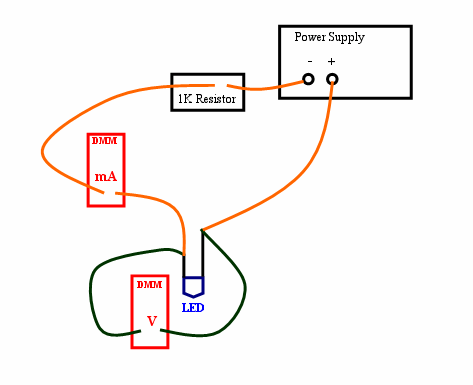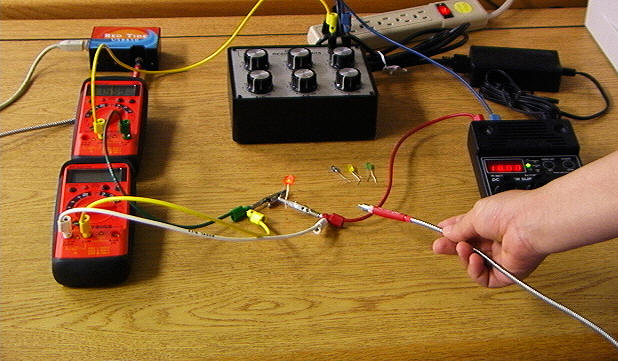• Wire the red LED in series with a 1 kΩ resistor and a DMM set to measure current in mA to the PASCO SF-9584A Low Voltage DC Power Supply as shown in the figure above.  Connect another DMM set to measure voltage in V directly across the LED.  Set the voltage control on the power supply to zero volts and turn ON the supply.
• Increase the power supply voltage very slowly.  You will notice that at a certain voltage, the LED begins to emit light.  Record the voltage at which light first appears in the spreadsheet.  Then record the voltage across the LED when the current through the circuit is 0.2 mA, 0.4  mA, ... .  Measure and record the voltage across the LED for all the current values listed in the spreadsheet.  Measure the peak wavelength of the light.  Record the wavelength in the spreadsheet.
• To measure the wavelength, connect the "red Tide" spectrometer to a USB port of your computer.  Bring the fiber close to the LED.  Use the Capstone program to measure intensity versus wavelength, and find the wavelength of the peak.
• Repeat the above measurements for the yellow, green and clear LEDs.
• Produce a graph of voltage (vertical axis) versus current (horizontal axis) for each LED.  Note the 'knee' in the curve around the voltage at which light first appears.  This voltage is a little less than the gap voltage Vg.  To determine the gap voltage more accurately construct a graph of voltage versus current using only data points for which the current is 6 mA or above.  Extrapolate this graph to zero current by fitting it with a trend line.  Display the equation on the graph.  The intercept at zero current is your best estimate for the gap voltage.  The width of the band gap is Eg = eVg.  Record this width in the spreadsheet
• The energy of a photon is hf = hc/λ.  Estimate the energy of the emitted photons using your measure peak wavelength λ for all the diodes and record it in the spreadsheet.
• Find the difference hc/λ - Eg.  This should be the photon energy supplied by thermal energy.

Quantum Dots

Since you have all the equipment out on the table, complete the Quantum Dots exercise.

Open Microsoft Word and prepare a report.

#### Laboratory 6 Report

• Summarize the experiment.